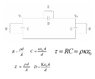# Leaky capacitor problem

dpd069

## Homework Statement

a. A leaky parallel plate capacitor has an area A. Its dielectric has thickness d, dielectric constant k(Kappa), and resistivity p(rho). Find its resistance R and its capacitance C, and its time constant for internal discharge t(tau).
b. A second leaky capacitor is mostly the same except it has dielectric constant K. Find its capacitance D and resistance Z.

c. Two capacitors of type (a.) and one of type (b.) are connected as shown (see attached picture). Write formulas for their charges and leak currents in terms of node voltages v1 and v2.
d. write Kirchoff's current law for each numbered node.

## The Attempt at a Solution

I believe i have solutions for parts a and b. I am struggling to figure out part c. The layout of the circuit is that of a pi-network and i have no idea how to follow the circuit from the node to write a formula for the capacitors charges and leak currents in terms of the node voltages.

#### Attachments

•Problem 3.jpg
14.4 KB · Views: 432